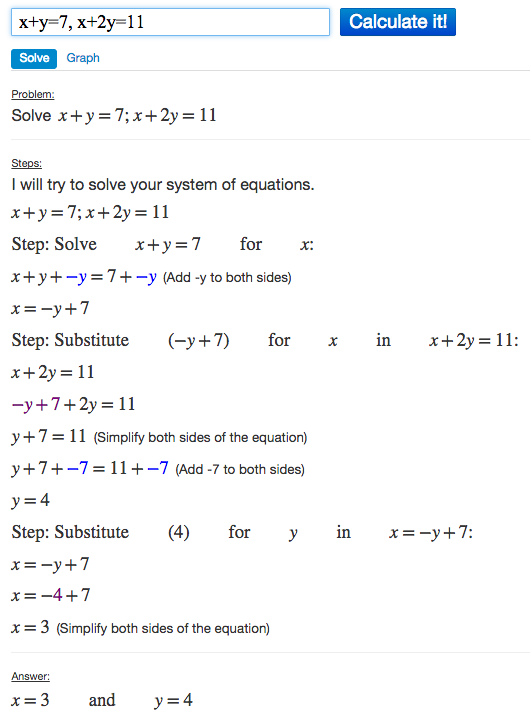# Solving Systems of Equations Using Algebra Calculator

Learn how to use the Algebra Calculator to solve systems of equations.

### Example Problem

Solve the following system of equations:
x+y=7, x+2y=11

### How to Solve the System of Equations in Algebra Calculator

First go to the Algebra Calculator main page.

Type the following:

1. The first equation x+y=7
2. Then a comma ,
3. Then the second equation x+2y=11
Try it now: x+y=7, x+2y=11

### Clickable Demo

Try entering x+y=7, x+2y=11 into the text box.After you enter the system of equations, Algebra Calculator will solve the system x+y=7, x+2y=11 to get x=3 and y=4.### More Examples

Here are more examples of how to solve systems of equations in Algebra Calculator. Feel free to try them now.